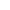# Question regarding fx-82EXBest Answer Tritonio , 16 September 2019 - 04:36 PM

Shift+= will not simply convert the number on screen to decimal representation. What it does is that it does the calculation on screen (in the last case Ans x 2) and shows the result of the calculation in decimal representation. So the first time you do Ans x 2 and you press =, the result gets stored in Ans. So when you then press Shift+= it will do the new Ans value, times two and show you that result in decimal.

Keep doing shift+= and you'll see that it keeps doubling it every time. The same thing that it would do if you kept pressing = after Ans x 2. It would keep doubling every time but it would show it as fraction.

Oh and to avoid it, use the S<->D button above the DEL button. This will convert the result from fraction to decimal and back to fraction (try pressing it many times) but it will do that without recalculating the expression you typed in.

Go to the full post3 replies to this topic

### #1Ossiak

Ossiak

Newbie

•• Members
•• 2 posts

• Calculators:
fx-82EX

Posted 16 September 2019 - 01:59 PM

This is the first time I'm using a relatively advanced calculator, and I have already run into a small problem.

Try for example:

4 divided by 3

Result = 4/3

Press shift+= and get the result in decimals (= 1.33333333)

Multiply this by 2

Result = 8/3

Press shift+= to get result in decimals

Result = 5.333333333

8 divided by 3 is obviously not 5.33333333, but rather half (2.666666667).

For some reason, while doing the operations above, it multiplies the result (8/3) again before showing it in decimals.

Am I missing something? Is there some way to avoid this? Is it a bug?

Thanks.

### #2Tritonio

Tritonio

•• Members
•• 77 posts
• Gender:Male

• Calculators:
fx-5800P, fx-991ES+, fx-991EX, HP Prime, fx-9750GIII, fx-3650P II

Posted 16 September 2019 - 04:36 PM   Best Answer

Shift+= will not simply convert the number on screen to decimal representation. What it does is that it does the calculation on screen (in the last case Ans x 2) and shows the result of the calculation in decimal representation. So the first time you do Ans x 2 and you press =, the result gets stored in Ans. So when you then press Shift+= it will do the new Ans value, times two and show you that result in decimal.

Keep doing shift+= and you'll see that it keeps doubling it every time. The same thing that it would do if you kept pressing = after Ans x 2. It would keep doubling every time but it would show it as fraction.

Oh and to avoid it, use the S<->D button above the DEL button. This will convert the result from fraction to decimal and back to fraction (try pressing it many times) but it will do that without recalculating the expression you typed in.

### #3Ossiak

Ossiak

Newbie

•• Members
•• 2 posts

• Calculators:
fx-82EX

Posted 17 September 2019 - 06:12 AM

That clarifies everything. Thank you very much for such an extensive answer!

### #4Tritonio

Tritonio

•• Members
•• 77 posts
• Gender:Male

• Calculators:
fx-5800P, fx-991ES+, fx-991EX, HP Prime, fx-9750GIII, fx-3650P II

Posted 17 September 2019 - 06:16 PM

No problem and welcome to the forum.

#### 0 user(s) are reading this topic

0 members, 0 guests, 0 anonymous users